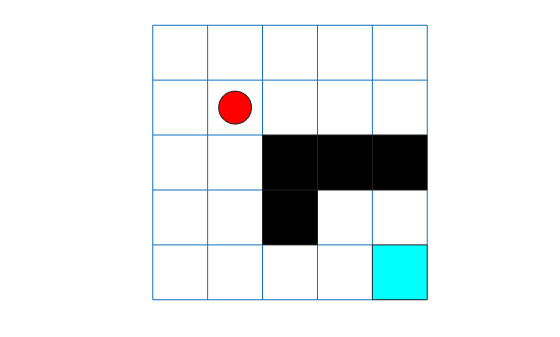# createGridWorld

Create a two-dimensional grid world for reinforcement learning

Since R2019a

## Syntax

``GW = createGridWorld(m,n)``
``GW = createGridWorld(m,n,moves)``

## Description

example

````GW = createGridWorld(m,n)` creates a grid world `GW` of size `m`-by-`n` with default actions of `['N';'S';'E';'W']`.```
````GW = createGridWorld(m,n,moves)` creates a grid world `GW` of size `m`-by-`n` with actions specified by `moves`.```

## Examples

collapse all

For this example, consider a 5-by-5 grid world with the following rules:

1. A 5-by-5 grid world bounded by borders, with 4 possible actions (North = 1, South = 2, East = 3, West = 4).

2. The agent begins from cell [2,1] (second row, first column).

3. The agent receives reward +10 if it reaches the terminal state at cell [5,5] (blue).

4. The environment contains a special jump from cell [2,4] to cell [4,4] with +5 reward.

5. The agent is blocked by obstacles in cells [3,3], [3,4], [3,5] and [4,3] (black cells).

6. All other actions result in -1 reward.First, create a `GridWorld` object using the `createGridWorld` function.

`GW = createGridWorld(5,5)`
```GW = GridWorld with properties: GridSize: [5 5] CurrentState: "[1,1]" States: [25x1 string] Actions: [4x1 string] T: [25x25x4 double] R: [25x25x4 double] ObstacleStates: [0x1 string] TerminalStates: [0x1 string] ProbabilityTolerance: 8.8818e-16 ```

Now, set the initial, terminal and obstacle states.

```GW.CurrentState = '[2,1]'; GW.TerminalStates = '[5,5]'; GW.ObstacleStates = ["[3,3]";"[3,4]";"[3,5]";"[4,3]"];```

Update the state transition matrix for the obstacle states and set the jump rule over the obstacle states.

```updateStateTranstionForObstacles(GW) GW.T(state2idx(GW,"[2,4]"),:,:) = 0; GW.T(state2idx(GW,"[2,4]"),state2idx(GW,"[4,4]"),:) = 1;```

Next, define the rewards in the reward transition matrix.

```nS = numel(GW.States); nA = numel(GW.Actions); GW.R = -1*ones(nS,nS,nA); GW.R(state2idx(GW,"[2,4]"),state2idx(GW,"[4,4]"),:) = 5; GW.R(:,state2idx(GW,GW.TerminalStates),:) = 10;```

Now, use `rlMDPEnv` to create a grid world environment using the `GridWorld` object `GW`.

`env = rlMDPEnv(GW)`
```env = rlMDPEnv with properties: Model: [1x1 rl.env.GridWorld] ResetFcn: [] ```

You can visualize the grid world environment using the `plot` function.

`plot(env)`## Input Arguments

collapse all

Number of rows of the grid world, specified as a scalar.

Number of columns of the grid world, specified as a scalar.

Action names, specified as either `'Standard'` or `'Kings'`. When `moves` is set to

• `'Standard'`, the actions are `['N';'S';'E';'W']`.

• `'Kings'`, the actions are `['N';'S';'E';'W';'NE';'NW';'SE';'SW']`.

## Output Arguments

collapse all

Two-dimensional grid world, returned as a `GridWorld` object with properties listed below. For more information, see Create Custom Grid World Environments.

Size of the grid world, specified as a `[m,n]` vector.

Name of the current state, specified as a string.

State names, specified as a string vector of length `m`*`n`.

Action names, specified as a string vector. The length of the `Actions` vector is determined by the `moves` argument.

`Actions` is a string vector of length:

• Four, if `moves` is specified as `'Standard'`.

• Eight, `moves` is specified as `'Kings'`.

State transition matrix, specified as a 3-D array, which determines the possible movements of the agent in an environment. State transition matrix `T` is a probability matrix that indicates how likely the agent will move from the current state `s` to any possible next state `s'` by performing action `a`. `T` is given by,

`T` is:

• A `K`-by-`K`-by-4 array, if `moves` is specified as `'Standard'`. Here, `K` = `m`*`n`.

• A `K`-by-`K`-by-8 array, if `moves` is specified as `'Kings'`.

Reward transition matrix, specified as a 3-D array, determines how much reward the agent receives after performing an action in the environment. `R` has the same shape and size as state transition matrix `T`. Reward transition matrix `R` is given by,

`R` is:

• A `K`-by-`K`-by-4 array, if `moves` is specified as `'Standard'`. Here, `K` = `m`*`n`.

• A `K`-by-`K`-by-8 array, if `moves` is specified as `'Kings'`.

State names that cannot be reached in the grid world, specified as a string vector.

Terminal state names in the grid world, specified as a string vector.

## Version History

Introduced in R2019a